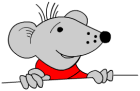# Free Y3 maths worksheet: counting on (teens)

Free maths worksheet from mathsblog.co.ukCounting on is a very powerful tool when using mental methods of calculating. This worksheet concentrates on counting on a teen number to a number in the hundreds and can be seen as an addition problem (143 + 16).

The usual method of doing this requires the ability to partition (ie know that 16 is 10 + 6) and is in two parts:

1. Count on ten from 143 making 153.

2. Count on 6 from 153 to make 159.

There should not be a need to do this type of question on paper.

Free maths worksheet: counting on (teens)